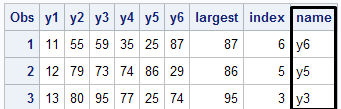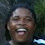# SAS : Find Variable with the Max or Min in a row

This tutorial demonstrates how to find a variable with the maximum or minimum value for each row (observation) in SAS. It's pretty straightforward to calculate max or min value but a little problematic to identify the variable name against the value.

Let's create Sample Data
input y1-y6;
cards;
11 55 59 35 25 87
12 79 73 74 86 29
13 80 95 77 25 74
;
run;
Find Variable Name Containing Max Value in a row
data out;
array values y1-y6;
largest = max(of values[*]);
index    = whichn(largest, of values[*]);
name = vname(values[index]);
proc print;
run;Output

Explanation :
1. array values y1-y6 : Lists all the variables for calculating max value
2. max() function calculates maximum value of all the variables listed in step 1 across rows
3. whichn() function returns the column index number of the matching value. In this case, it is searching the maximum value across rows and returns the column position in the listed variables. For example, it returns 6 in row 1 as 87 is the maximum value in row 1 and it is placed at 6th column of y1-y6
4. vname() function returns the variable name. In this case, it calculates variable name of the largest value.

To Find Variable Name containing minimum value
Use min() function instead of max() in the code above and the remaining code would be same.

Find Top 3 Variables

Suppose you are asked to identify top 3 variables across rows. You can use largest function in SAS.

LARGEST Function : Syntax
largest(k, variables)
k : kth value you want (2 for the second largest value)
data want;
array values[*] y1-y6;
array large;
array names \$32;
do i = 1 to dim(large);
large[i] = largest(i,of values[*]);
index    = whichn(large[i],of values[*]);
names[i] = vname(values[index]);
end;
drop i index;
proc print;
run;SAS : LARGEST values

Explanation :
1. array values[*] y1-y6  -  Specify all the variables from which you want to calculate top 3 variables
2. array large - Top 3 large values
3. array names \$32 - Names of top 3 variables
4. do i = 1 to dim(large) -  3 iterations for calculating first, second and third largest values
5. large[i] = largest(i,of values[*]) - largest value when i =1, second largest when i =2 and so on.
6. index = whichn(large[i],of values[*]) : Column index for kth largest values
7. names[i]    = vname(values[index]) :  Extract the variable name of the largest using largest index
8. drop i index; : Dropping Irrelevant Variables

Find Bottom 3 Variables
Refer the code above and change largest() function to smallest() function.
Related Posts
ShareDeepanshu founded ListenData with a simple objective - Make analytics easy to understand and follow. He has over 10 years of experience in data science. During his tenure, he worked with global clients in various domains like Banking, Insurance, Private Equity, Telecom and HR.

3 Responses to "SAS : Find Variable with the Max or Min in a row"
1.I found this very useful

2.Your post is interesting - thank you for publishing it. Unfortunately, the algorithm is not very robust. Let's suppose that some of your values are missing or that two or more values are identical within an observation. You'll see it is not working properly. I tried to find a correction but unfortunately, I cannot.

Best regards,

Jean Hardy
SAS Consultant and trainer
1.